Physics Primer

Physics Primer

This physics primer will take you throughthe solution of a basic kinematics problem.

Racing on the Highway

A car is racing on the highway. At themoment t=0 it passes by a police car. The police car starts chasing the racingcar at the moment t=t1 and it reaches the racing at the moment t=t2.The velocity of the racing is v, and the velocity of the police car is u. Wewill assume that the cars are always moving with constant speed. We will tackletwo questions:

If we know the velocity of the police car(u), and the time when it starts the chase (t1) and the time when itreaches the racing (t2), what is the velocity of the racing car (v)?

If we know the velocity of the police car(u), the velocity of the racing car (v) and the time when the police car startsthe chase (t1), what is the time (t2) when the police carreaches the racing car?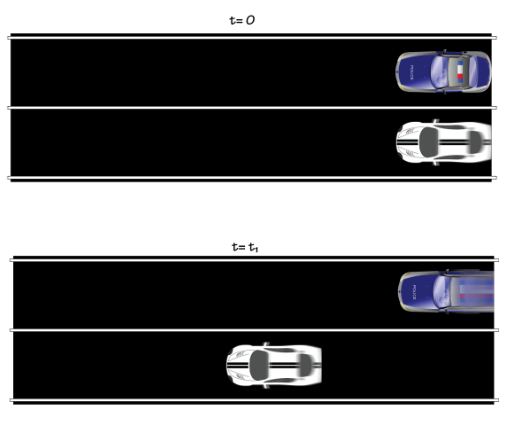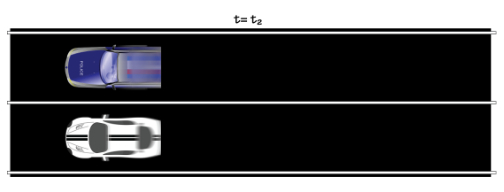Solution

When tackling such a problem don’t startwith writing down formulas. Instead, think about the best way of relating therelevant quantities. What connects them? The answer to this question will neverbe a formula, but a physical insight in the problem.

In the case of our problem, the keyobservation is to notice that the police car and the racing car are in the sameplace at the time t=0 and the time t=t2. This means that in the timet2 they both covered the same distance.

Distance covered by racing car in the timet2=Distance covered by police car in the time t2

Now we just need to relate this distance tothe velocity of the two cars. The racing car is moving at a constant speedthroughout the time t2, so it will cover a distance:

D=vt2

The police car only starts moving at thetime t1. That means that it only moves for the time t2−t1.For this period of time its speed is constant, so it will cover a distance:

D=u(t2−t1)

But we know that both cars cover the samedistance, so

vt2=u(t2−t1)

This is the equation that solves both ourquestions. If we want we can determine v, or t2: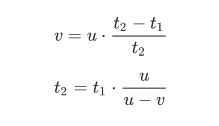Verification

The best practice is to always check youranswers. This implies not only double checking the algebraic manipulations, butchecking especially the soundness of the physical answer. Most often you willspot mistakes by realizing that your answer doesn’t make physical sense.

Dimensions

The golden rule of dimensional analysisrequires that dimensions on the left must match dimensions on the right. Noticethat each fraction has no dimensions because it is a ratio of two quantitieswith the same dimension (time over time, velocity over velocity). So only theterm in front matters in terms of dimensions. Fortunately we got a velocity onboth sides, and a time on both sides.

Physical Intuition

A solution must make physical sense.  Because the police car catches up with theracing car, it is clear that it must move faster than the racing car. That is,v<u. This is true because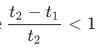. Inthe case of the time solution, we must check that our answer is positive: u−v>0,all good here. And that t2>t1, which is true because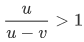.

When typing the solution I mistakenly switchedu and v – this is an easy to make mistake because there is no sign to pointwhich velocity corresponds to which car. I spotted the mistake when checking ifmy answer made sense.